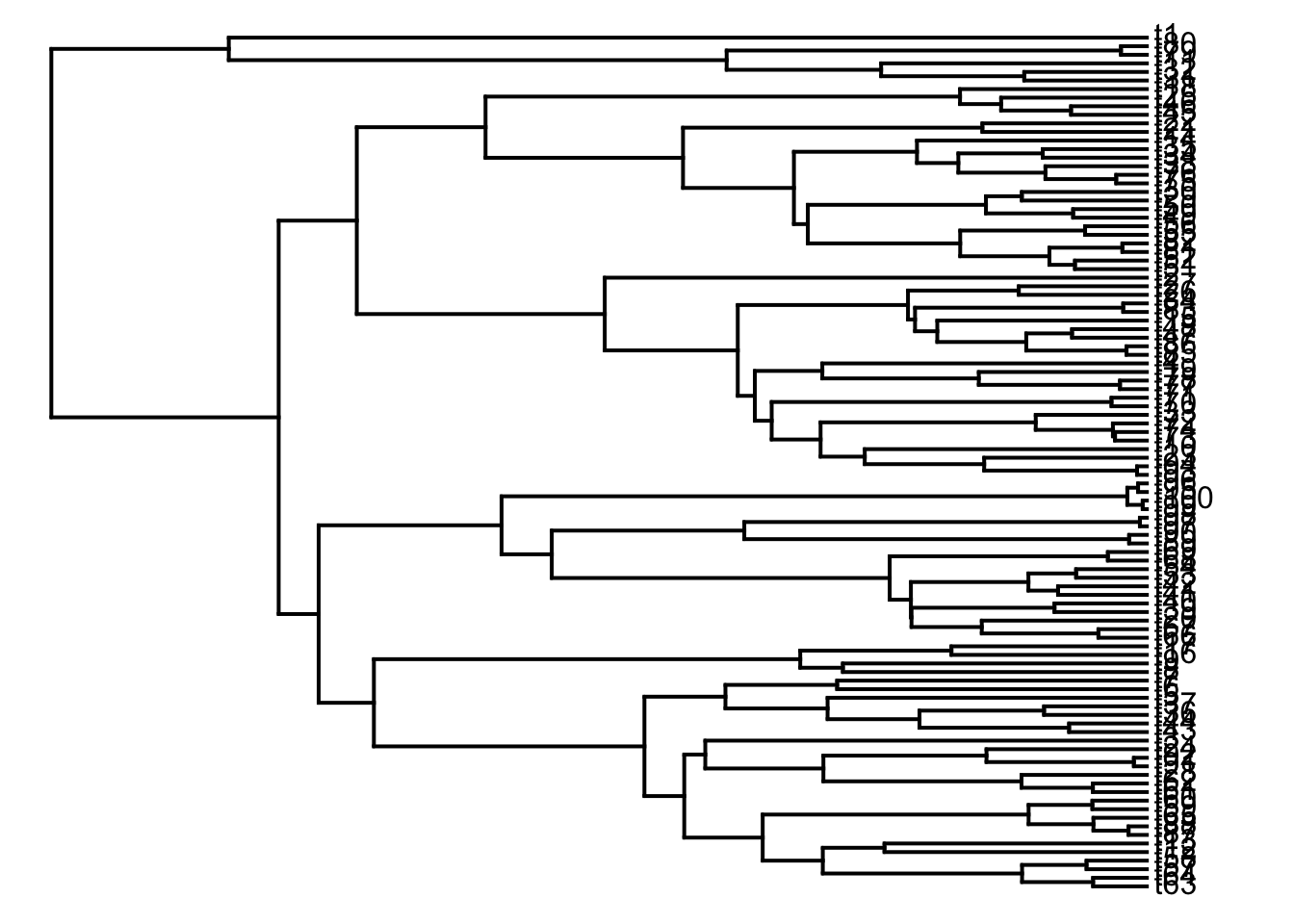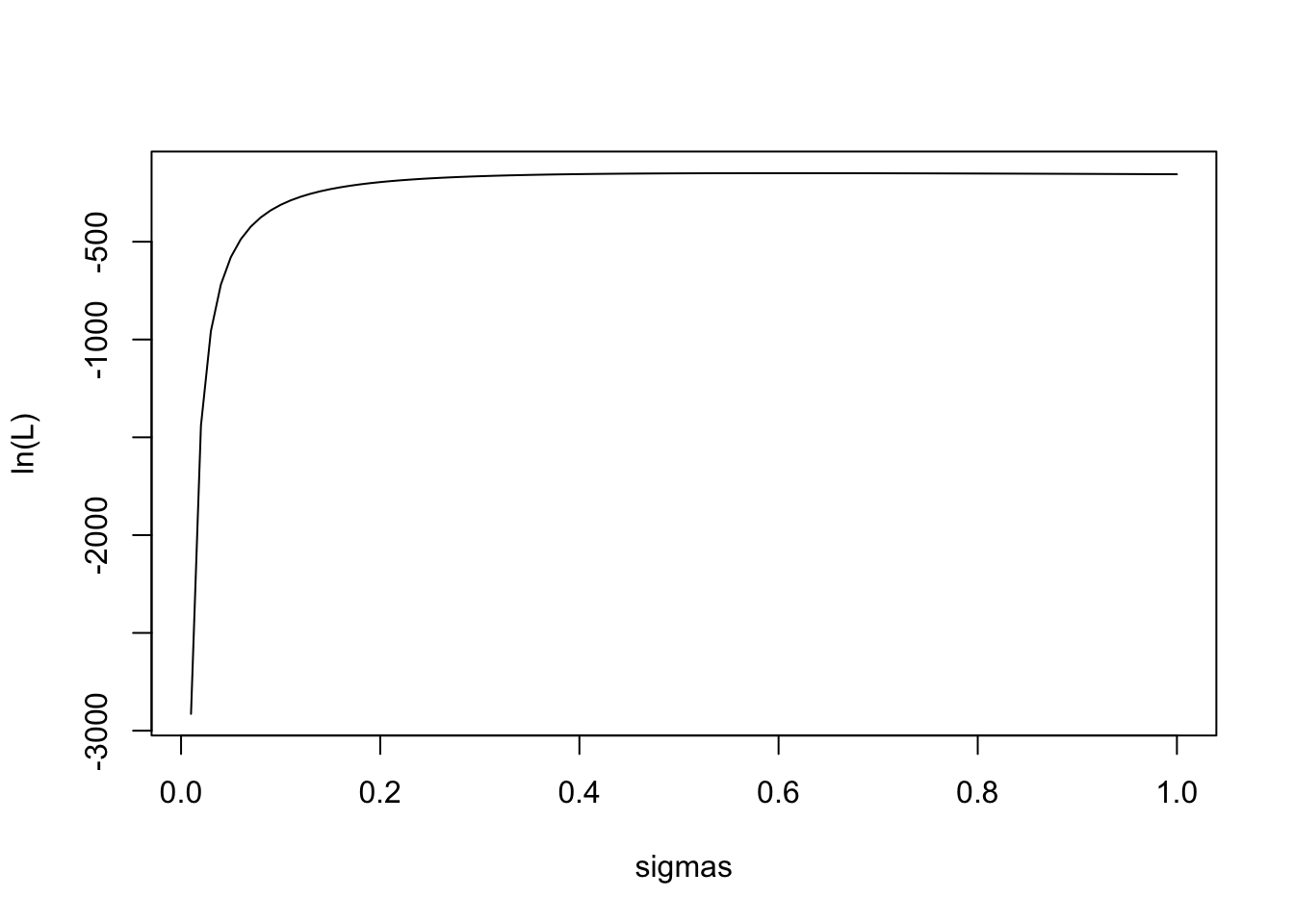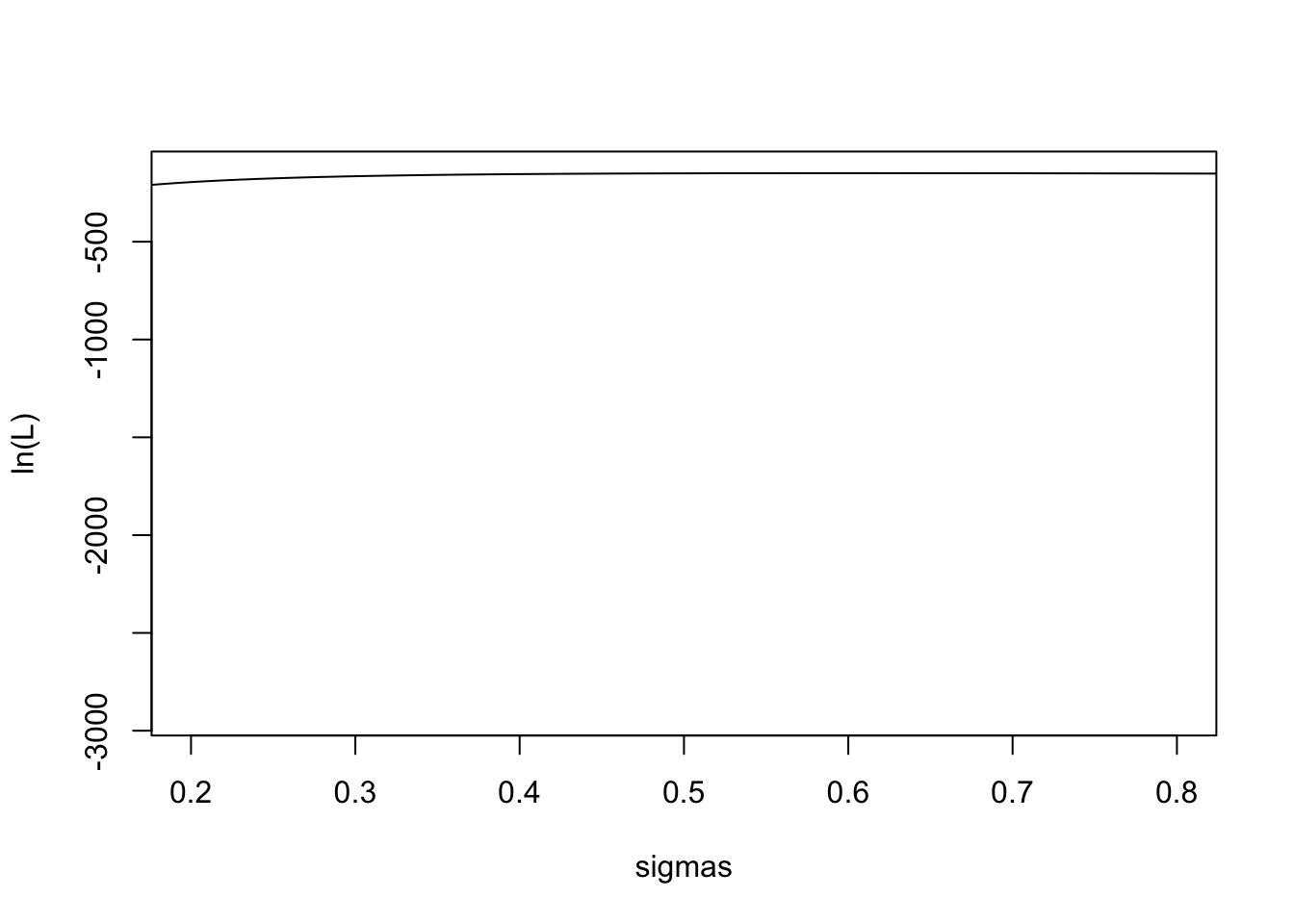Here are a few more details about Brownian motion

BMlk is function that calculates the likelihood of BM. There are two steps to follow here .

1. We can get the ML estimate of sigma^2 following the equation from O’Meara 2006.

### Eq. 1

$\hat{\sigma}^2 = \frac{[X - E(X)]'C^{-1}[X- E(X)]}{N}$

Where $$X$$ is a vector of the tip values, $$E(X)$$ is the expected values at the root, $$C$$ is the phylogenetic variance-covariance matrix, and $$N$$ is the number of tips. Also…

• $$[X - E(X)]'$$ is the transpose of the tip values minus the root value
• $$C^{-1}$$ is the inverse of the phylogenetic variance-covariance matrix
1. We can get the likelihood of any value of $$\sigma^2$$ by using equation 1:

### Eq. 2

$log(L) = log\left [ \frac{exp\left \{ -\frac{1}{2}[X - E(X)]'V^{-1}[X- E(X)] \right \}}{((2\pi)^N*det(V)} \right ]$

$$V$$ here is called the rate matrix. It is simply the proposed value of $$\sigma^2$$ multiplied by the phylogenetic variance-covariance matrix. So, Eq. 2 is saying that the likelihood (or rather the log of the likelihood) is a function of the difference between the tip and root values and the rate matrix, $$V$$ (which is simply the vcv matrix multiplied by some value of $$\sigma^2$$ that we wish to evaluate).

We use can use Eq 2 to write a function in R that will allow us to compare likelihoods given some tree and and a $$\sigma^2$$. Our function will take the following arguments…

• the phylogenetic variance-covariance matrix
• the inverse of the vcv matrix
• $$\sigma^2$$
• the root state, and
• a vector of the tips values for the trait
require(phytools)
## Loading required package: phytools
## Loading required package: ape
## Loading required package: maps
##
##  # maps v3.1: updated 'world': all lakes moved to separate new #
##  # 'lakes' database. Type '?world' or 'news(package="maps")'.  #
BMlk <- function(C, inv.C, sigmasq, root.state, data) {

N <- length(data); # the number of tips
EX <- rep(root.state, N) # creates a vector of the expected trait value - which under BM is the root state
V <- C * sigmasq; # multiply the entries in C by the BM rate
inv.V <- inv.C * sigmasq ^-1; # do the same for the inverted matrix using the inverse of the rate

lnlNum<- -0.5*(data - EX) %*% inv.V %*% (data - EX)
lnlDen<- log(sqrt((2*pi)^N*det(V)))
L<-lnlNum-lnlDen
return(L);
}

Note that we are going to have to do a little work before we can use our function. We need to pass it a vcv matrix and the inverse of the matrix, we need to pass in a root state value, and we need to pass a value of $$\sigma^2$$. Let’s try a fast example. We will…

1. simulate a 5 taxon tree
2. simulate BM on that tree with a known $$\sigma^2$$ of 0.5
3. compare the likelihood of the generating $$\sigma^2$$ to a smaller $$\sigma^2$$ of 0.005.
# we can check this works for a really simple example - let's simulate a tree and some data and try it out.
require(TreeSim);
## Loading required package: TreeSim
## Loading required package: laser
## Loading required package: geiger
require(phytools);

t <- 10  # total time
n <- 100  # total taxa
b <- (log(n) - log(2))/t
tree <- pbtree(b = b, n = n, t = t)
## simulating with both taxa-stop (n) and time-stop (t) is
## performed via rejection sampling & may be slow
##
##   99 trees rejected before finding a tree
data <- fastBM(tree, sig2 = 0.5);
plotTree(ladderize(tree))#data
# we'll need the covariance matrix
c <- vcvPhylo(tree, anc.nodes = F);
#c
inv.c <- solve(c);

Lets compare the likelihoods of the generating sigma square and a much lower value

#inv.c

## now we'll compute the likelihood of the true value and a different value ##

BMlk(c, inv.c, sigmasq = 0.5, root.state = 0, data  = data); # the true value for sigsq
##           [,1]
## [1,] -150.7476
#BMlk(c, inv.c, sigmasq = 0.05, root.state = 0, data  = data); #
BMlk(c, inv.c, sigmasq = 0.005, root.state = 0, data  = data); # 
##           [,1]
## [1,] -5899.365

Remember when comparing likelihoods, the higher the number, the better the likelihood (because the likelihood of a hypothesis is proportional to the probability of the data given that the hypothesis is true). Smaller (more negative) log likelihoods are also smaller likelihoods (raise $$e$$ to the power of the competing likelihoods to check this) so we can see that our data prefers the the generating $$\sigma^2$$ value to the alternative.

We can also look at the likelihood surface around the generating value

vals <- rep(0,100)
sigmas <- 1:100/100
thetas <- 1:100/1000
for(ii in 1:100){

vals[ii] <- BMlk(c, inv.c, sigmasq = sigmas[ii], root.state = sigmas[ii], data  = data)
}
vals
##    -2913.9403 -1438.7831  -955.7866  -718.5371  -578.7152  -487.1794
##     -422.9934  -375.7506  -339.7030  -311.4221  -288.7390  -270.2161
##    -254.8642  -241.9808  -231.0539  -221.7016  -213.6338  -206.6262
##    -200.5028  -195.1237  -190.3762  -186.1688  -182.4263  -179.0865
##    -176.0975  -173.4156  -171.0037  -168.8305  -166.8689  -165.0957
##    -163.4909  -162.0369  -160.7186  -159.5225  -158.4370  -157.4516
##    -156.5572  -155.7455  -155.0093  -154.3421  -153.7381  -153.1920
##    -152.6992  -152.2552  -151.8564  -151.4992  -151.1804  -150.8972
##    -150.6469  -150.4271  -150.2357  -150.0705  -149.9299  -149.8120
##    -149.7154  -149.6385  -149.5801  -149.5389  -149.5138  -149.5037
##    -149.5077  -149.5249  -149.5545  -149.5956  -149.6475  -149.7096
##    -149.7812  -149.8618  -149.9508  -150.0476  -150.1519  -150.2631
##    -150.3808  -150.5047  -150.6344  -150.7695  -150.9097  -151.0547
##    -151.2042  -151.3579  -151.5157  -151.6771  -151.8421  -152.0104
##    -152.1818  -152.3562  -152.5332  -152.7128  -152.8948  -153.0791
##    -153.2655  -153.4539  -153.6441  -153.8360  -154.0296  -154.2247
##    -154.4211  -154.6189  -154.8179  -155.0180
#filled.contour(sigmas, sigmas, vals)
plot(sigmas, vals,type = "l", ylab = "ln(L)")plot(sigmas,vals, xlim = c(0.2, 0.8), type = "l", ylab = "ln(L)")# BMlk(c, inv.c, sigmasq = 0.5, root.state = 0, data  = data); # the true value for sigsq
# #BMlk(c, inv.c, sigmasq = 0.05, root.state = 0, data  = data); #
# BMlk(c, inv.c, sigmasq = 0.49, root.state = 0, data  = data); #
# BMlk(c, inv.c, sigmasq = 0.48, root.state = 0, data  = data); # 

The likelihood surface is quite flat around the generating value!

Another look at contrasts andBM rate…

library(phytools)
## simulate a coalescent shaped tree
tree<-rcoal(n=50)
plot(tree,ftype="off")
## Warning in plot.window(...): "ftype" is not a graphical parameter
## Warning in plot.xy(xy, type, ...): "ftype" is not a graphical parameter
## Warning in title(...): "ftype" is not a graphical parameter